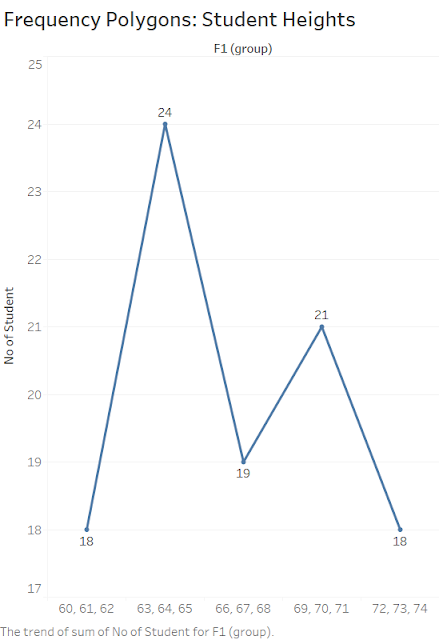Showing posts with label Distribution. Show all posts
Showing posts with label Distribution. Show all posts

## Wednesday, 31 July 2019

### Frequency Distribution #2 - #UnlockStats

Starting from the point where we left off - Frequency Distribution #1 - #UnlockStats
Below is the table for 100 students and their heights categories -
 Height (in) No of Students 60-62 5 63-65 18 66-68 38 69-71 31 72-74 8 total 100#### Histogram:

It consists of a set of rectangles having based on a horizontal axis with center at the class mark and width equal to the class intervals size and length proportional to class frequency.

The histogram shows how the data is distributed, In our example, the width is 3 of each category and left-skewed. Most of the data is left side of the histogram

####Frequency Polygon:

A Frequency Polygon is line graph of the class frequencies plotted against class marks = ( UCL + LCL ) / 2
It can be obtained by connecting the midpoint of the tops of the rectangles in the histogram.#### Box Plots:

A box plot shows a box which contains the middle 50% of data values, It also shows two whiskers that extend from the box to maximum and minimum value.

#### Relative Frequency Distribution:

The Relative Frequency of a class is the frequency of the class divided by total frequency of all the classes (total no of data points) and expressed in percentage.
 Height (in) Relative Frequency Distribution (%) 60-62 5 63-65 18 66-68 38 69-71 31 72-74 8

#### Cumulative Frequency Distribution:

The total frequency of all values less than the upper-class boundary of a given class interval is called the cumulative frequency up to and including that class interval.
 Height (in) No of Students Cum. Freq. Distribution 60-62 ( <=62) 5 5 63-65 (<=65) 18 5+18 = 23 66-68 (<=68) 38 23 + 38 = 16 69-71 (<=71) 31 61 + 31 = 92 72-74 (<=74) 8 92 + 8 = 100

A line plot between Upper-Class Boundary and Cum. Frequency is called Cum Freq Distribution polygon or ogive.

#### Cumulative Relative Frequency Distribution:

 Height (in) No of Students Cum. Rel. Freq. Distribution (%) <=62 5 5 <=65 18 23 <=68 38 16 <=71 31 92 <=74 8 100

23% of the students have less than or equal to 65 inches.

#### Types of Frequency Curves:

a. Symmetrical or bell curves are characterized by the fact that observations equidistance from the central maximum has the same frequency.
b. Curves that have tails to the left are said to be skewed to the left.
c. Curves that have tails to the right are said to be skewed to the right.
d. Curves that have approx equal frequencies across their values are said to be uniformly distributed.
e. J-shaped or reverse J-shaped frequency curve the maximum occurs at one end or the other.
f. A U-shaped curve has maxima at both end and minimum in between.
g. A bimodal frequency curve has two maxima.
h. A multimodal frequency curve has more than 2 maxima.

Like the below page to get the update
Facebook Page      Facebook Group      Twitter Feed      Telegram Group

## Tuesday, 30 July 2019

### Frequency Distribution #1 - #UnlockStats

#### Raw Data:

Raw data are collected data that have not been organized in any way.#### Array:

An array is a list of raw numerical data in ascending or descending order of magnitude.

#### Frequency Distribution:

When summarizing large no of data, we categorized them into classes or categories and no of individuals belongs to each class is called the Class Frequency.
A tabular arrangement of data by classes or categories with class frequency is called Frequency Distribution.

#### Example:

Below is the table for 100 students and their heights categories -

 Height (in) No of Students 60-62 5 63-65 18 66-68 38 69-71 31 72-74 8 total 100

#### Class Intervals and Class Limits:

A symbol defining a class is called Class Intervals such as 63-65, also called Closed Class Intervals as Class has end numbers.
The end no of the class is called Class Limits such as 66 and 68 where 66 is Lower Class Limit and 68 is Upper-Class Limit.  If Class has either no upper class nor no lower class is called an Open Class Intervals such as category 65+years.

#### Class Boundaries:

Class Boundaries can be defined by adding upper-class limit if a category to lower class limit of the next category by 2.

Upper-Class Boundary (n) - { UCL(n) + LCL(n+1) } / 2

For 63-65 category, 65.5 { (65+66)/2 } is upper-class limit and 62.5 { (62+63)/2 } is lower class limit.

#### Size/Width of a Class Interval:

The difference between the lower and upper-class limit is called size or width of a Class Interval.
such as -
For 63-65 category, Width is - 65.5 - 62.5 = 3

#### The Class Mark:

The Class Mark is mid-point of a Class interval and can be calculated as below -

Class Mark (n) - { UCL(n) + LCL(n) } / 2

For 63-65 category, Class Mark is - (63 + 65) / 2 = 64

Histogram and Frequency Polygon are two graphic representation of frequency distribution. We will discuss this more in the next post.

Till then, Happy Learning.........

Like the below page to get the update
Facebook Page      Facebook Group      Twitter Feed      Google+ Feed      Telegram Group